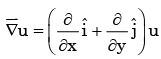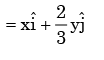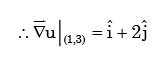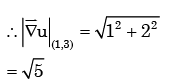Courses

# Partial Derivatives, Gradient - MCQ Test - 2

## 25 Questions MCQ Test Topicwise Question Bank for Electronics Engineering | Partial Derivatives, Gradient - MCQ Test - 2

Description
This mock test of Partial Derivatives, Gradient - MCQ Test - 2 for GATE helps you for every GATE entrance exam. This contains 25 Multiple Choice Questions for GATE Partial Derivatives, Gradient - MCQ Test - 2 (mcq) to study with solutions a complete question bank. The solved questions answers in this Partial Derivatives, Gradient - MCQ Test - 2 quiz give you a good mix of easy questions and tough questions. GATE students definitely take this Partial Derivatives, Gradient - MCQ Test - 2 exercise for a better result in the exam. You can find other Partial Derivatives, Gradient - MCQ Test - 2 extra questions, long questions & short questions for GATE on EduRev as well by searching above.
QUESTION: 1

### f(x, y) = x2 + xyz + z Find fx at (1,1,1)

Solution:

fx = 2x + yz

Put (x,y,z) = (1,1,1)

fx = 2 + 1 = 3.

QUESTION: 2

### Eight people are planning to share equally the cost of a rental car. If one person withdraws from the arrangement and the others share equally the entire cost of the car, then the share of each of the remaining persons increased by:

Solution: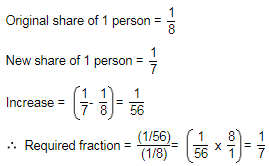QUESTION: 3

### The minimum point of the function f(x) = (x2/3) – x is at

Solution:

Correct Answer :- a

Explanation : f(x) = (x^2/3) - x

f'(x) = 2/3(x-1/2) - 1

f"(x) = -1/3(x-3/2)

For critical points. f′(x)=0

=> 2/3(x-1/2) - 1 = 0

f has minimum value of x = 1

QUESTION: 4

If x=a(θ+ sin θ) and y=a(1-cosθ), then dy/dx will be equal

Solution: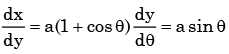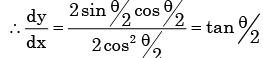QUESTION: 5

The minimum value of function y = x2 in the interval [1, 5] is

Solution:

y =x 2 is strictly increasing function on [1,5]

∴ y= x 2 has minimum value at x = 1 is 1.

QUESTION: 6

The function f(x) = 2x3 – 3x2 – 36x + 2 has its maxima at

Solution: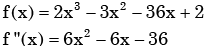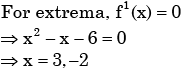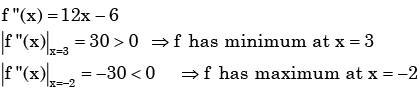QUESTION: 7

What should be the value of λ such that the function defined below is continuous at x = π/22?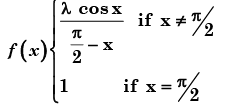Solution:

By the given condition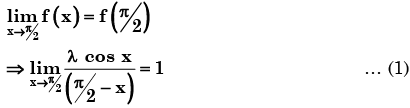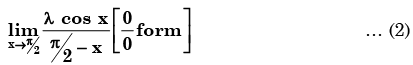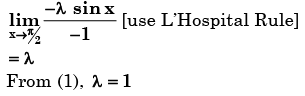QUESTION: 8

Consider function f(x) =(x2-4)2 where x is a real number. Then the function has

Solution: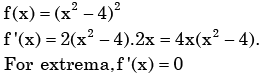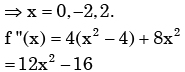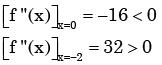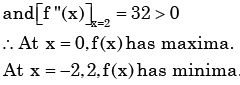QUESTION: 9

If f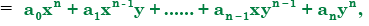where ai (i = 0 to n) are constants, then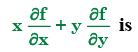Solution: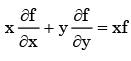- Euler’s theorem for homogeneous function

QUESTION: 10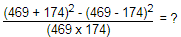Solution: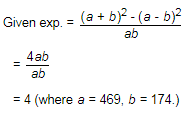QUESTION: 11

A point on a curve is said to be an extremum if it is a local minimum or a local maximum. The number of distinct exterma for the curve 3x4 – 16x3 – 24x2 + 37 is

Solution: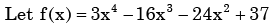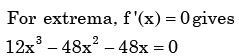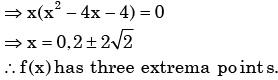QUESTION: 12

∇ × ∇ × P, where P is a vector, is equal to

Solution:
QUESTION: 13

The value of the integral of the function g(x, y) = 4x3 + 10y4 along the straight line segment from the point (0, 0) to the point (1, 2) in the x-y plane is

Solution:

The equation of the line passing through (0,0) and (1,2)  is y = 2x

Given y x, y ) = 4x3+ 10y4 = 4x3 + 10(2x )4 = 4x3+ 160xy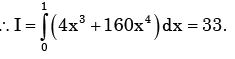QUESTION: 14

If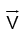is a differentiable vector function and f is a sufficient differentiable scalar function, then curl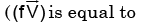Solution: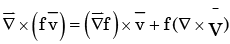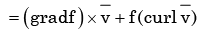QUESTION: 15

The temperature field in a body varies according to the equation T(x,y) = x3+4xy. The direction of fastest variation in temperature at the point (1,0) is given by

Solution: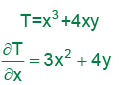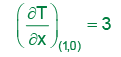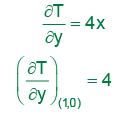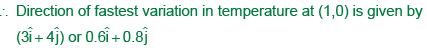QUESTION: 16

The divergence of vector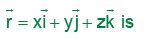Solution: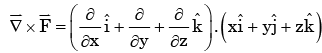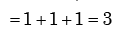QUESTION: 17

The divergence of the vector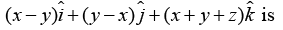Solution:
QUESTION: 18

Among the following, the pair of vectors orthogonal to each other is

Solution: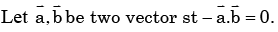Then we say that they are orthogonal.  Choice (c) is correct.

QUESTION: 19

The directional derivative of the scalar function f(x, y, z) = x2 + 2y2 + z at the point P = (1,1, 2) in the direction of the vector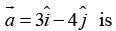Solution:

Required directional derivatives at P(1,1,-1)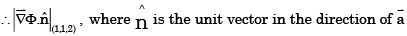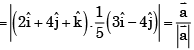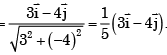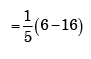=2

QUESTION: 20

The Gauss divergence theorem relates certain

Solution:
QUESTION: 21

If P, Q and R are three points having coordinates (3, –2, –1), (1, 3, 4), (2, 1, –2) in XYZ space, then the distance from point P to plane OQR (O being the origin of the coordinate system) is given by

Solution:

The equation of the plane OQR is (O being origin).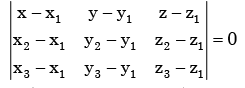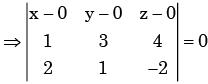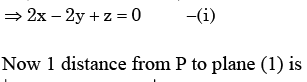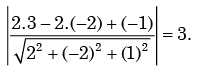QUESTION: 22

Let x and y be two vectors in a 3 dimensional space and <x, y> denote their dot product.

Then the determinant detSolution: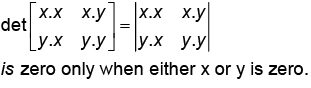QUESTION: 23

If a - b = 3 and a2 + b2 = 29, find the value of ab.

Solution:

2ab = (a2 + b2) - (a - b)2

= 29 - 9 = 20ab = 10.

QUESTION: 24

If a vector R(t)  has a constant magnitude, then

Solution: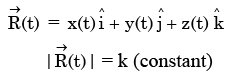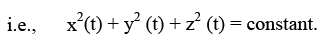On analysing the given (a) option, we find that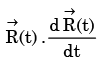will give constant magnitude, so first
differentiation of the integration will be zero.

QUESTION: 25

For the scalar field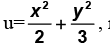magnitude of the gradient at the point(1,3) is

Solution: